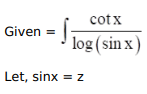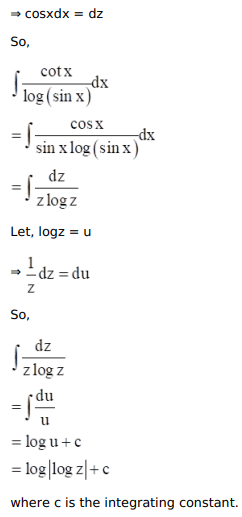# Mark against the correct answer in each of the following:

Question:

Mark $(\sqrt{)}$ against the correct answer in each of the following:

$\int \frac{\cot x}{\log (\sin x)} d x=?$

A. $\log |\cot x|+C$

B. $\log |\cot x \operatorname{cosec} x|+C$

C. $\log |\log \sin x|+C$

D. none of these

Solution: